Evaluation

The reconstructed geometry of the D&M Pyramid shows a five-sided object having facets with differing angles. The object has bilateral symmetry, with a pair of congruent angles forming the front, and another pair of congruent angles forming the sides. In the illustration below, the arrow points along the axis of bilateral symmetry.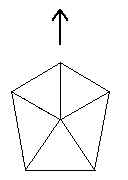The D&M Pyramid appears to be positioned with architectural alignment to other enigmatic objects nearby that have also been studied as possibly artificial. The main axis of the D&M as illustrated above points at the Face in Cydonia. Henceforth we will refer to this direction as the "front" of the pyramid.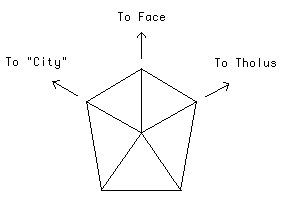The front of the D&M Pyramid has three edges, spaced 60 degrees apart. As noted above, the center axis points to the Face. The edge on the left of this axis points toward the center of a feature that has been nicknamed the "City" by the Cydonia investigators. The edge on the right of the center axis points toward the apex of a dome-like structure known as the "Tholus".

The five-sidedness, bilateral symmetry, and primary alignments were first observed by Richard Hoagland after studying quality digital enlargements prepared in 1984 by SRI International from negatives of images processed by DiPietro and Molenaar. These events are documented in detail by Hoagland  and Pozos .

Turning back to the reconstructed geometry, we will now consider the internal symmetries of this object.

The D&M Pyramid displays a complex interplay between five-fold and six-fold symmetry. Both symmetries are present simultaneously, with the front of the pyramid exhibiting six-fold symmetry, and the "ground level" of the pyramid yielding a 36 degree angle that is characteristic of five-fold symmetry.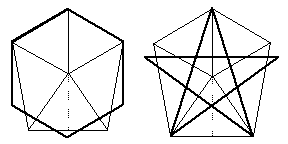It is worth noting that the practice of combining symmetries was widely practiced by the architects of antiquity. It was believed that geometry and certain mathematical relationships were crucial building blocks of the Cosmos, and that architecture should reflect these symmetries. These practices were later revived in the Islamic world and especially in Renaissance Europe.

The angles formed by the D&M Pyramid when viewed from above differ from each other. Consequently, they can form various ratios. These angle ratios were studied to see if the values were significant, or merely random.

The angle ratios display significant values, with a preponderance of square roots and fractions involving square roots. Once again, we have a theme used by Classical architects, who used the square roots of two, three, and five in laying out the proportions of their buildings.

For clarity, three of these angle ratios are illustrated below: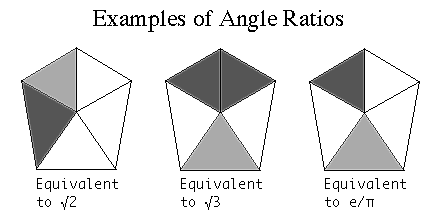The ratios of the shaded angles are equivalent to the indicated values. In the right hand illustration, the ratio of the shaded angles is equivalent to the ratio of e (the base of the natural logarithms) and pi (the relationship between a circle's diameter and circumference).

(This ratio of e/pi is nearly equal to the square root of three divided by two, and the precision of measurement, +/- 0.2 deg, is insufficient to distinguish between these two possible values.)

A table has been prepared displaying a list of the measured angles, and the results of the analysis. The square roots of three and five, and the values of e and pi predominate. The identity of these values is strengthened by the numerous combinations in which they occur.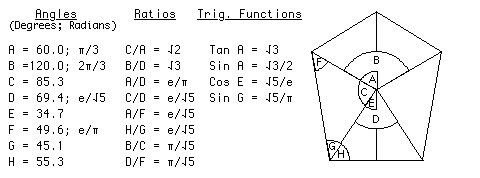Note that the radian measure and trigonometric functions of some angles yield the same values produced by the angle ratios. The geometry thus has a common contextual thread.

As mentioned earlier in the section on criteria, all of this geometry is "dimensionless", i.e. it is not dependent on such cultural conventions as counting by tens, or measuring angles in the 360 system. This geometry will "work" in any number system.

A Speculation Concerning Tetrahedral Geometry

The aforementioned ambiguity concerning e/pi vs. the square root of three divided by two can be resolved with the geometry of a circumscribed tetrahedron.

A tetrahedron is the simplest of the Platonic solids, a polyhedron having six edges, four vertices, and four sides where each side is an equilateral triangle. A circumscribed tetrahedron is a tetrahedron that has been enclosed in a sphere that just touches each of the four vertices.

The presence of 60 degree angles produces the ambiguity: the Sine of 60 degrees is defined as (sqrt 3)/2, and this is very close, but not equal to, the ratio of e/pi:

(sqrt 3)/2 = 0.866025
e/pi = 0.865256

It is this ambiguity that is resolved by the geometry of a circumscribed tetrahedron.

The surface area of a sphere, divided by the surface area of the tetrahedron circumscribed by it, yields a very close approximation of e which shall be termed e':

e = 2.718282
e' = 2.720699

When the ratio e/pi is evaluated using this "tetrahedral approximation of e", the result is precisely equal to (sqrt 3)/2:

e/pi = 0.865256
e'/pi = 0.866025 = (sqrt 3)/2

Conclusions

This investigation of the D&M Pyramid reveals a morphology that is inconsistent with the surrounding geology. The geomorphological processes observed to exist on Mars not only fail to provide a potential mechanism for the D&M Pyramid's formation, but seem to preclude its very existence. Analysis of the object's geometry, and its alignment with other anomalous landforms, reveal intricate relationships that are numerous and logical, and are suggestive of highly sophisticated design.

This object has been compared with the elaborate symbolic architecture of antiquity. While much of the geometry is the same utilized by Classical architects, it is important to note that the implementation is totally different. Nowhere in Earth history is this exact type of geometric symbolism to be found.

The same techniques used for most of this century in air photo interpretation show that the D&M Pyramid may be artificial, or may be a natural landform modified by intelligence. The true nature of this object can be resolved by re-imaging the Cydonia region to obtain high-resolution imagery of the D&M Pyramid and the other enigmatic landforms nearby. The issue of possible ruins at Cydonia is thus the only question involving extraterrestrial intelligence that can be easily resolved with known scientific means.

The author wishes to acknowledge Dr. Mark J. Carlotto, The Analytic Sciences Corporation, the National Space Science Data Center for the imagery and coordinate information, and Mr. Richard C. Hoagland for background information and collaboration on the research described herein.